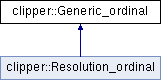Clipper
clipper::Generic_ordinal Class Reference

Generic ordinal gernerator. More...

`#include <clipper_stats.h>`

Inheritance diagram for clipper::Generic_ordinal:List of all members.

## Public Member Functions

Generic_ordinal ()
null constructor
Generic_ordinal (const Range< ftype > &range, const int &n)
constructor: from range and sampling
void init (const Range< ftype > &range, const int num_ranges=1000)
initialiser: takes the source range and sampling
void init (const std::vector< ftype > &values, const int num_ranges=1000)
initialiser: takes the source distibution and a number of bins
ftype ordinal (const ftype &value) const
return reflection ordinal
void accumulate (const ftype &value)
accumulate values to build the distribution
void accumulate (const ftype &value, const ftype &weight)
accumulate values to build the distribution
void prep_ordinal ()
generate the ordinal histogram
void invert ()
invert distribution to get value from ordinal
void init (const int num_ranges=1000)
DEPRECATED: initialiser: takes a number of bins for histogram.
void add_pass_1 (const ftype &value)
DEPRECATED: add a value to the distribution (pass 1 of 2)
void add_pass_2 (const ftype &value)
DEPRECATED: add a value to the distribution (pass 2 of 2)

## Protected Attributes

ftype nranges
number of ranges
Range< ftyperange_
resolution range of data
std::vector< ftypehist
histogram of reflections vs resolution

## Detailed Description

Generic ordinal gernerator.

This is a generic fast ordinal calculator. It is supplied with a list of values, from which it prepares a cumulative distribution function. This may the used to return the approximate fracitonal ordinal (in the range 0...1) for any given value from the distibution.

The distibution may be initialised by providing a vector of values from the distribution, or by adding the values and calling prep_ordinal().

This distribution may also be inverted. Generation of a value from an ordinal may be used for generating random values from a given distribution, or for histogram matching.

The documentation for this class was generated from the following files: